Question

# AH° = ? 3.(5pts) Consider the thermochemical reaction: CIF(g) + F (g) ----> CIF3(g) Use the...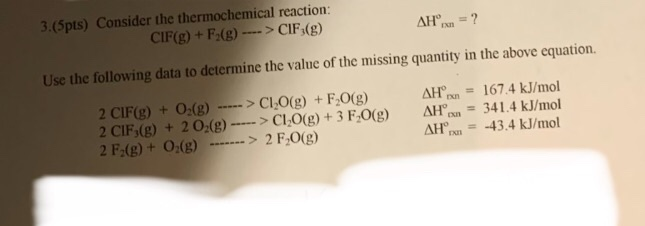AH° = ? 3.(5pts) Consider the thermochemical reaction: CIF(g) + F (g) ----> CIF3(g) Use the following data to determine the value of the missing quantity in the above equation. 2 CIF(g) + O2(g) -----> CI2O(g) + F2O(g) 2 CIF,(g) + 2 O2(g) ----> CI-O(g) + 3 F2O(g) 2 F2(g) + O2(g) -- -> 2 F.0(g) AH AH AH = 167.4 kJ/mol = 341.4 kJ/mol = -43.4 kJ/mol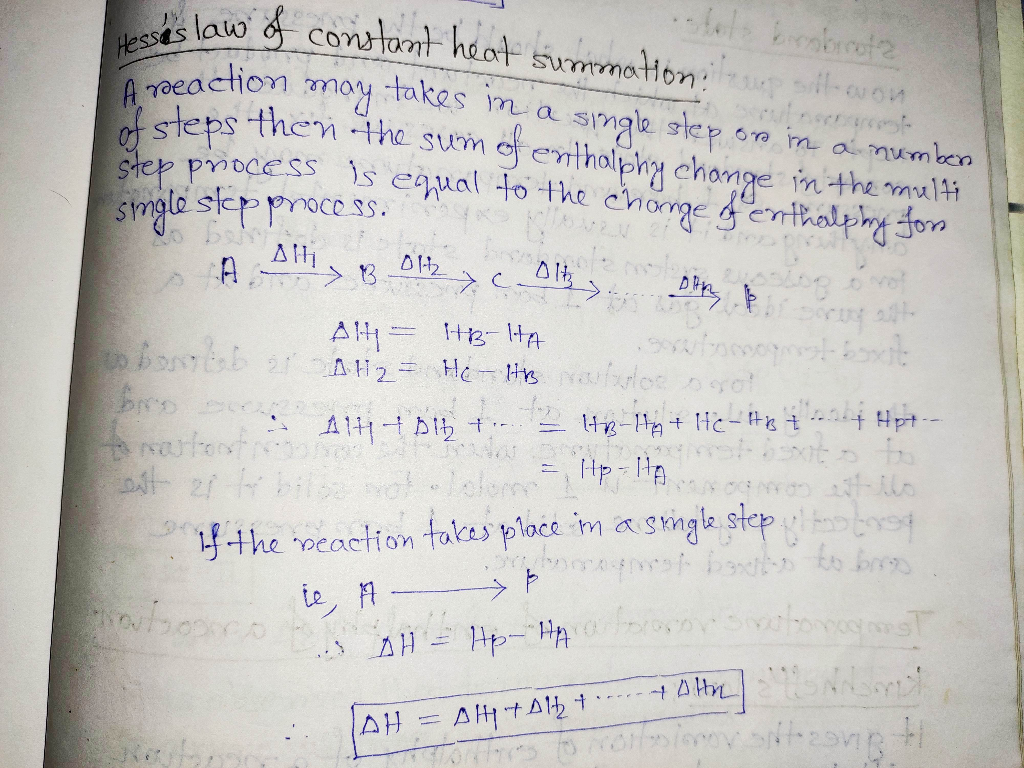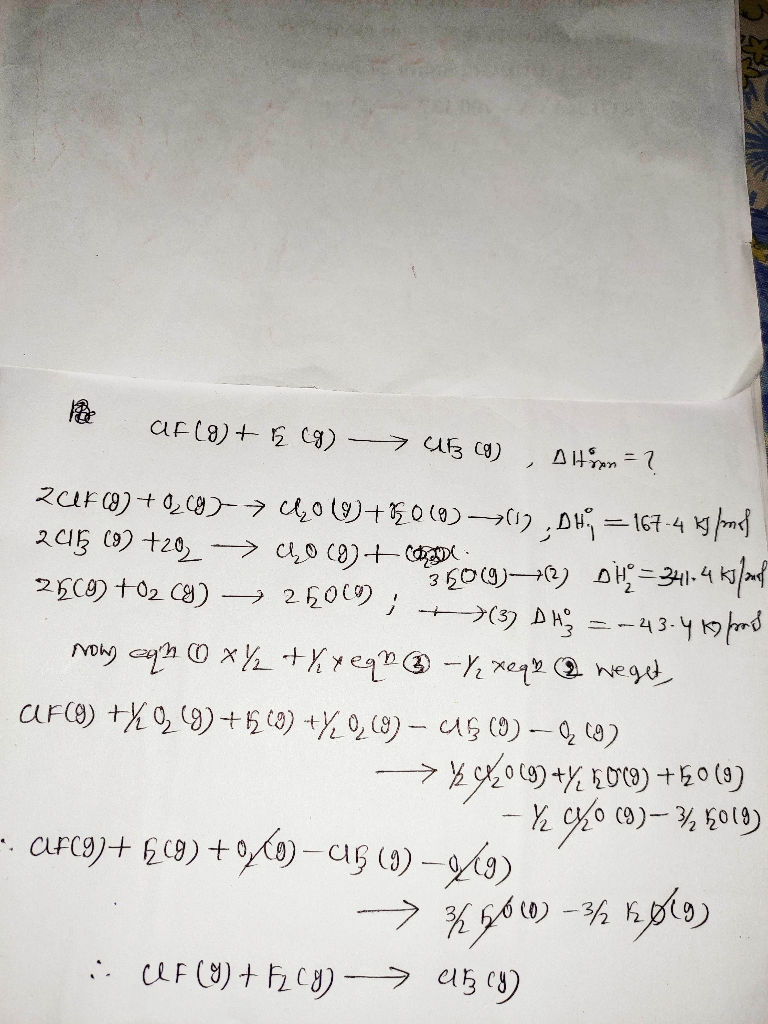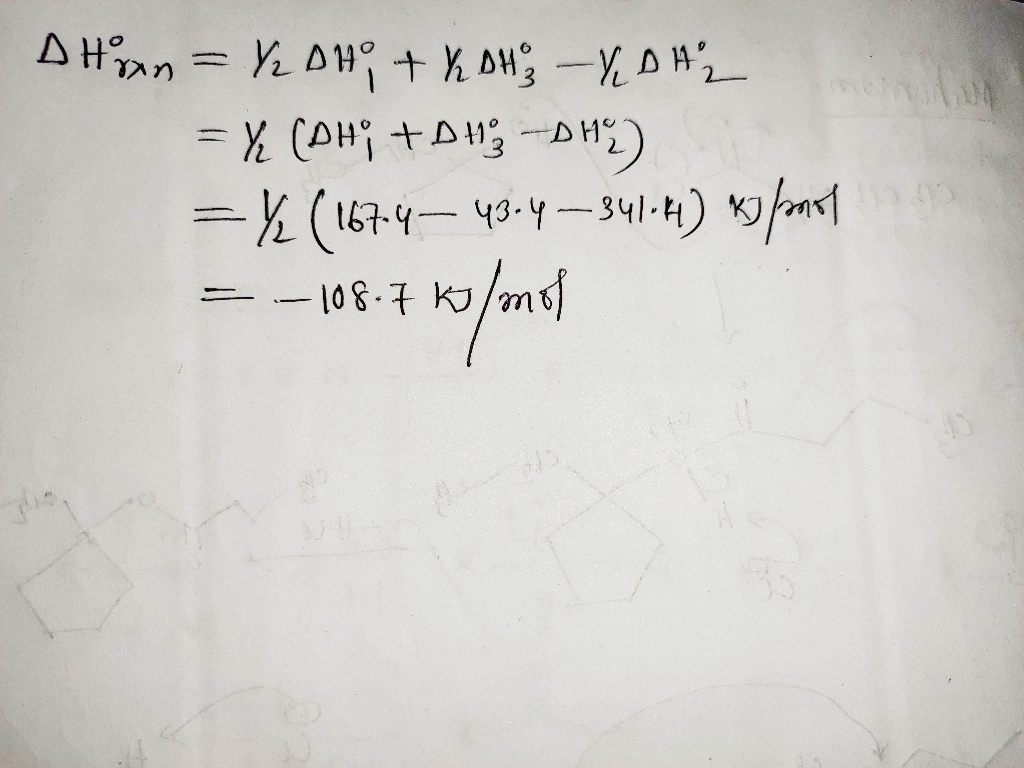#### Earn Coins

Coins can be redeemed for fabulous gifts.

Similar Homework Help Questions
• ### 3.(5pts)) Consider the thermochemical reaction: 3 CIF(g) + 3F.(g) ---->3 CIF,(g) AHºrn = ? Use the...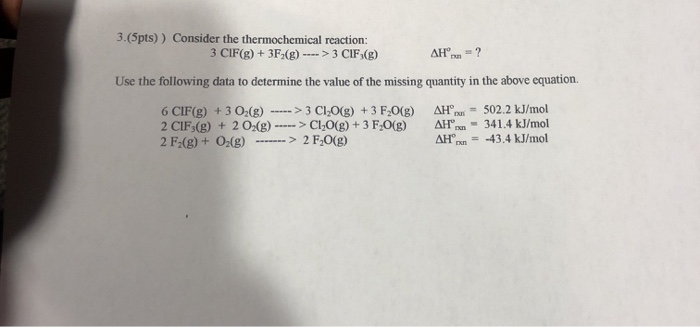3.(5pts)) Consider the thermochemical reaction: 3 CIF(g) + 3F.(g) ---->3 CIF,(g) AHºrn = ? Use the following data to determine the value of the missing quantity in the above equation. 6 CIF(g) + 3 O2(g) ----->3 ClO(g) + 3 F 0(g) 2 CIF3(g) + 2 02(8) -----> CLO(g) + 3 F.0() 2 F2(g) + O2(g) -------> 2 F.O(g) AH AH° AH - 502.2 kJ/mol 341.4 kJ/mol = -43.4 kJ/mol

• ### At 25°C, the following heats of reaction are known: 2CIF(g) + O2(g) → Cl2O(g) + F2O(g)...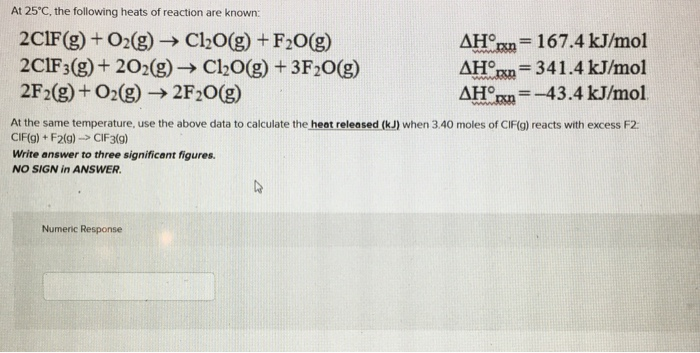At 25°C, the following heats of reaction are known: 2CIF(g) + O2(g) → Cl2O(g) + F2O(g) AHºrn = 167.4 kJ/mol 2C1F3(g) + 202(g) + Cl2O(g) + 3F2O(g) AHºrn = 341.4 kJ/mol 2F2(g) + O2(g) → 2F20(g) AHºrxn=-43.4 kJ/mol At the same temperature, use the above data to calculate the heat released (kJ) when 3.40 moles of CIF(g) reacts with excess F2. CIF(g) + F219) -> CIF3(g) Write answer to three significant figures. NO SIGN in ANSWER. Numeric Response

• ### 9. Given the following data: 2C1F(g) + O2(g) → Cl2O(g) + F20(g) 2C1F3(g) + 2O2(g) → Cl2O(g) + 3F20 2F2(g) + O2(g)...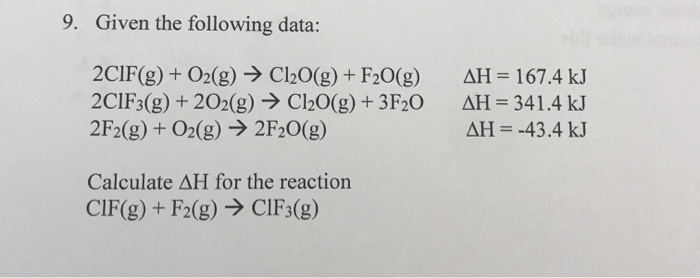9. Given the following data: 2C1F(g) + O2(g) → Cl2O(g) + F20(g) 2C1F3(g) + 2O2(g) → Cl2O(g) + 3F20 2F2(g) + O2(g) → 2F20(g) AH = 167.4 kJ AH = 341.4 kJ AH = -43.4 kJ Calculate AH for the reaction CIF(g) + F2(g) → CIF3(g) 10. Calculate AHº for the following reaction using the AHⓇ information below. 4NH3(g) + 5O2(g) → 4NO(g) + 6H2O(g) AH NH3(g) is -80 kJ/mol AH° NO(g) is 90 kJ/mol AH®, H2O(g) is -242 kJ/mol

• ### pter 4-6 0 Saved Help Save At 25°C, the following heats of reaction are known: 2C1F(g)...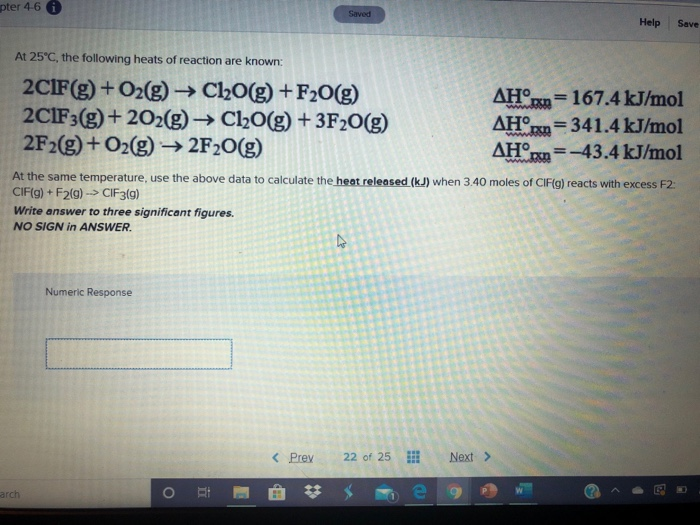pter 4-6 0 Saved Help Save At 25°C, the following heats of reaction are known: 2C1F(g) + O2(g) → Cl2O(g) +F20(g) 2C1F3(g) + 2O2(g) + Cl2O(g) + 3F2O(g) 2F2(g) + O2(g) → 2F20(g) AHO/= 167.4 kJ/mol AHºrn = 341.4 kJ/mol AHºrn=-43.4 kJ/mol At the same temperature, use the above data to calculate the heat released (kJ) when 3.40 moles of CIF(g) reacts with excess F2. CIF(g) + F2(9) --> CIF3(9) Write answer to three significant figures. NO SIGN in ANSWER....

Given the following data:2 ClF (g) + O2 (g) ? Cl2O (g) + F2O (g) ?H = 167.4 kJ2 ClF3 (g) + 2 O2 (g) ? Cl2O (g) + 3 F2O (g) ?H = 341.4 kJ2 F2 (g) + O2 (g) ? 2 F2O (g) ?H = -43.4 kJCalculate the ?H for the reactionClF (g) + F2 (g) ? ClF3 (g)

• ### At 25°C, the following heats of reaction are known: 2CIF(g) + O2(g) → Cl20(g) + F2。dH'm...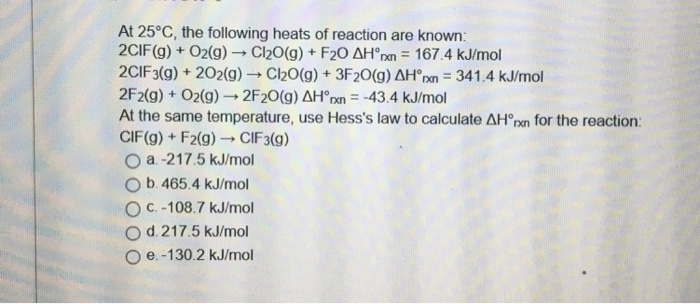At 25°C, the following heats of reaction are known: 2CIF(g) + O2(g) → Cl20(g) + F2。dH'm = 167.4 kJ/mol 2ClF3(g) + 202(g) → Cl20(g) + 3F20(g) ΔH'm-34 1.4 kJ/mol 2F2(g) + O2(g) → 2F20(g) ΔHoxn--43.4 kJ/mol At the same temperature, use Hess's law to calculate ΔHorn for the reaction: CIF(g) + F2(g) → ClF3(g) 0 a-21 7.5 kJ/mol O b 465.4 kJ/mol O c. -108.7 kJ/mol d 217.5 kJ/mol O e-130.2 kJ/mol

• ### At 25°C, the following heats of reaction are known: AH (kJ/mol 167.4 2CIF + 02 →Cl20...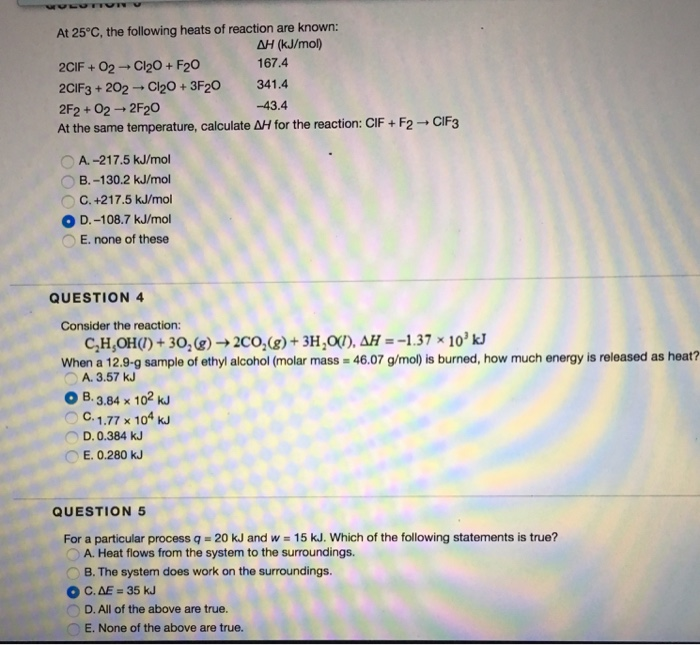At 25°C, the following heats of reaction are known: AH (kJ/mol 167.4 2CIF + 02 →Cl20 + F20 2ClF3 + 202 →Cl20+3F20 341.4 2F2 + 02 → 2F20 At the same temperature, calculate ΔH for the reaction: ClF + F2 → CIF3 -43.4 A. -217.5 kJ/mol B.-130.2 kJ/mol C. +217.5 kJ/mol ○ D.-108.7 kJ/mol E. none of these QUESTION 4 Consider the reaction: When a 12.9-g sample of ethyl alcohol(molar mass 46.07 g/mol) is burned, how much energy is released...

• ### 1.(3pts) Use the following data to determine the AH... of 2 CH2O(1) + 15 O_() ---> 10 CO(g) + 12 H.O(1) Data: Su...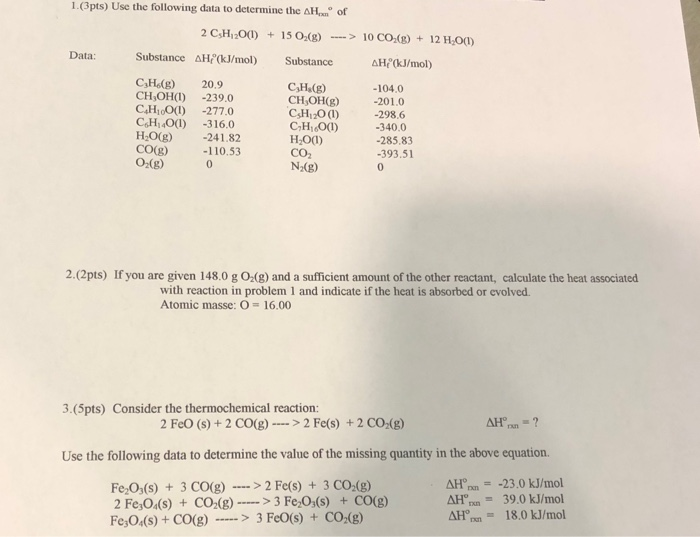1.(3pts) Use the following data to determine the AH... of 2 CH2O(1) + 15 O_() ---> 10 CO(g) + 12 H.O(1) Data: Substance AH(kJ/mol) Substance AH(kJ/mol) CHg) CH,OH(L) C.H.O(1) CH401) H2O(g) CO(g) O2(g) 20.9 -239.0 -277.0 -316,0 -241.82 -110.53 CH.(8) CH,OH(S) CsH 20 (1) CHO(1) HO() -104.0 -201.0 -298.6 -340.0 -285.83 -393.51 CO2 NB) 2.(2pts) If you are given 148.0 g O(g) and a sufficient amount of the other reactant, calculate the heat associated with reaction in problem 1 and...

• ### UL. Use the following data to determine AHºfor P.(s) + 5 CIF3(g) ----> 4 PF3 (g)...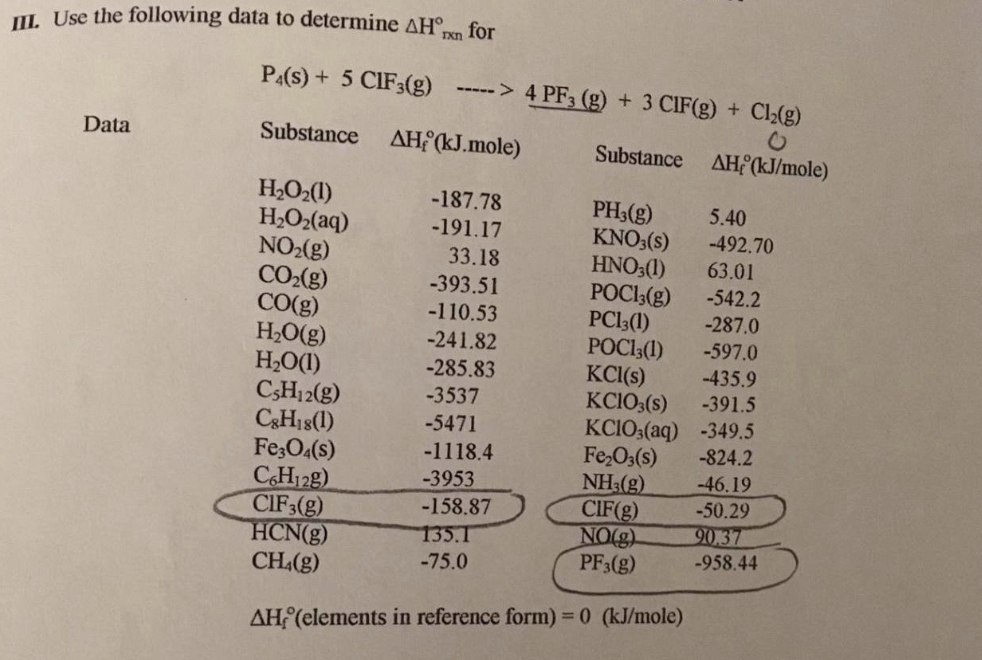UL. Use the following data to determine AHºfor P.(s) + 5 CIF3(g) ----> 4 PF3 (g) + 3 CIF(g) + Cl2(g) Data Substance AH°(kJ.mole) Substance AH°(kJ/mole) H2O2(1) H2O2(aq) NO2(g) CO2(g) CO(g) H2O(g) H2O(1) C3H2(g) C3H18(1) Fe3O4(s) C6H129) CIF3(g) HCN(g) CH (g) -187.78 -191.17 33.18 -393.51 -110.53 -241.82 -285.83 -3537 -5471 -1118.4 -3953 -158.87 135.1 -75.0 PH3(g) 5.40 KNO3(s) -492.70 HNO3(1) 63.01 POC13(g) -542.2 PC13(1) -287.0 POC13(1) -597.0 KCl(s) -435.9 KCIO3(s) -391.5 KCIO3(aq) -349.5 Fe2O3(s) -824.2 NH3(g) -46.19 CIF(g) -50.29 NO(g)...

• ### 1.(3pts) Use the following data to determine the AHıxn of 2 C3H12O(l) + 15 O2(g) ---->...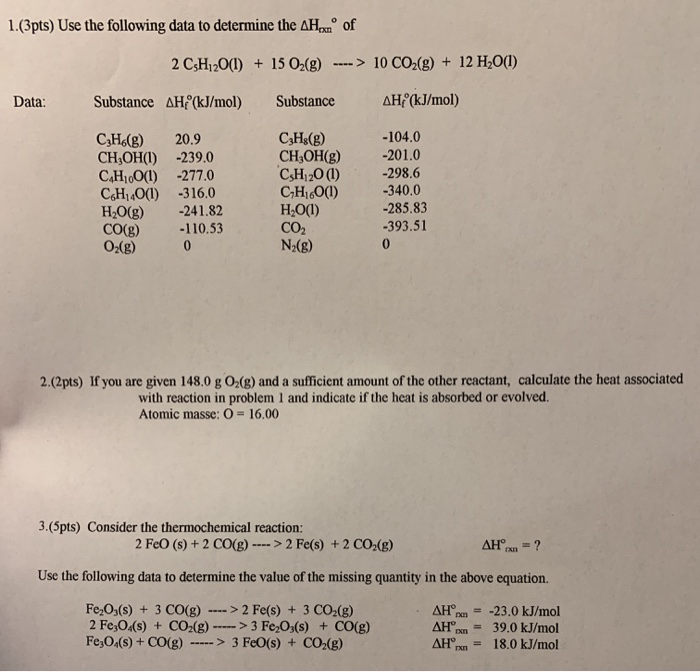1.(3pts) Use the following data to determine the AHıxn of 2 C3H12O(l) + 15 O2(g) ----> 10 CO2(g) + 12 H2O(1) Substance AH(kJ/mol) Substance AH(kJ/mol) Data: CzH.(g) CH,OH(1) C.H.0(1) C6H40(1) H2O(g) CO(g) O2(g) 20.9 -239.0 -277.0 -316.0 -241.82 -110.53 C3H2(g) CHOH(9) CH 20(1) CH;60(1) H2O(1) -104.0 -201.0 -298.6 -340.0 -285.83 -393.51 CO2 N (8) 2.(2pts) If you are given 148,0 g O2(g) and a sufficient amount of the other reactant, calculate the heat associated with reaction in problem 1 and...